### Home > CALC > Chapter 10 > Lesson 10.1.8 > Problem10-80

10-80.
1. Examine the integrals below. Consider the multiple tools available for integrating and use the best strategy. After evaluating each integral, write a short description of your method. Homework Help ✎

1.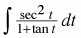2.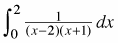3.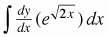4.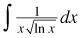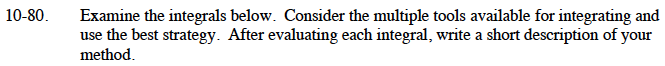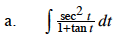Use substitution. Let u = tan(t).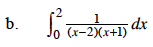Use partial fraction decomposition.

1/(x – 2) is undefined when x = 2, so a limit needs to be used to evaluate this integral.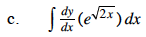Since this is the integral of a derivative, your answer should have a "+ C".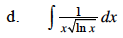Use substitution. Let u = ln(x).